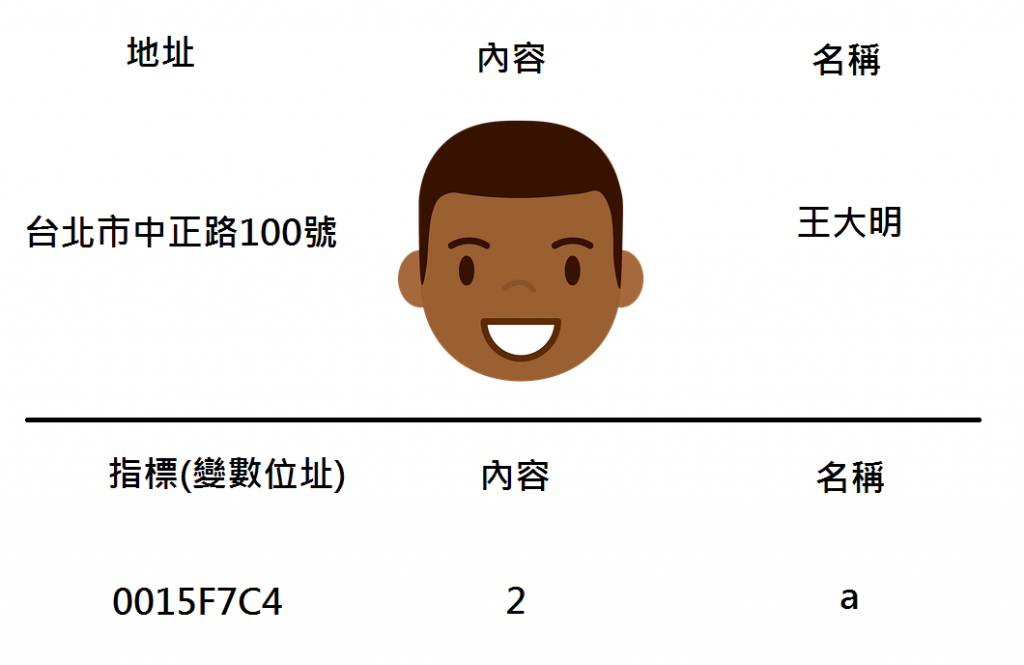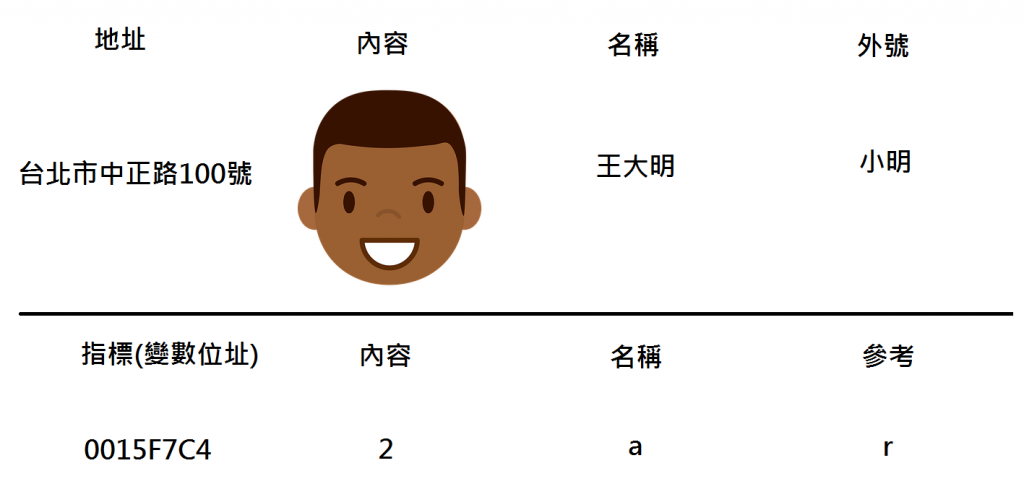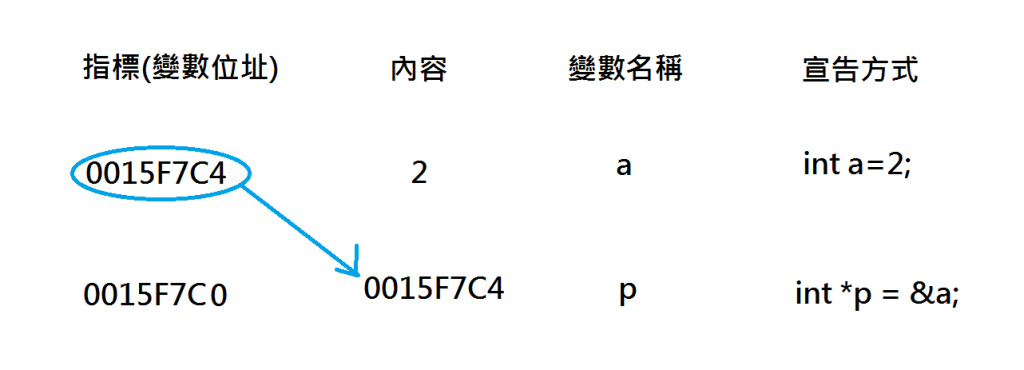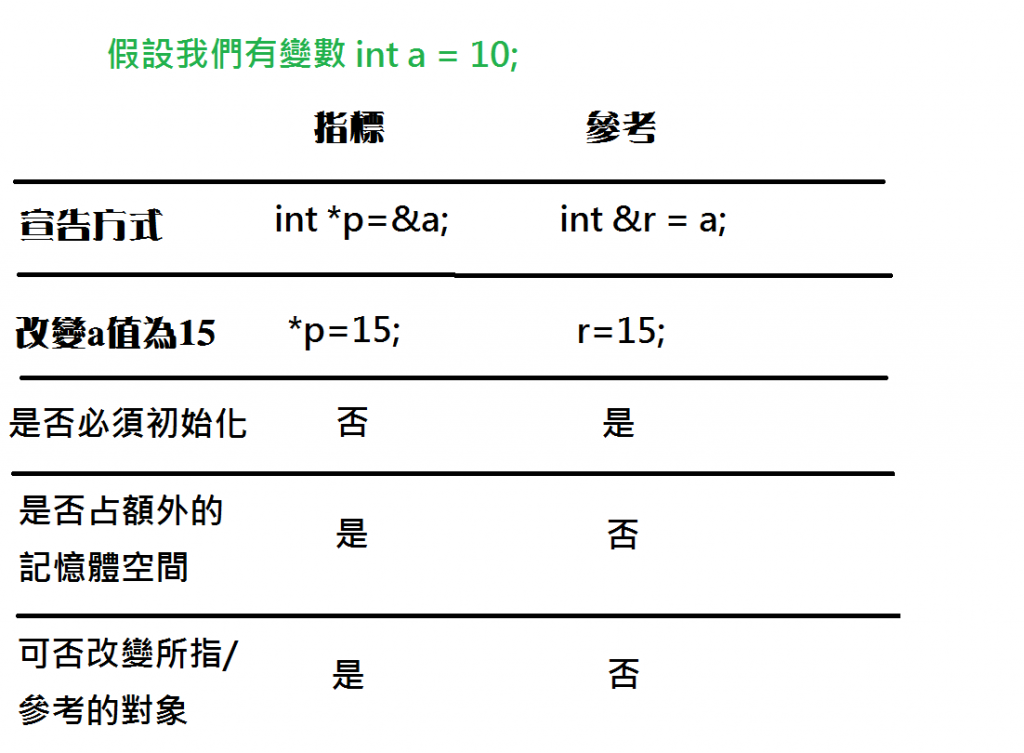5

# 觀念一、什麼是指標?``````void main(){
int a = 2;
}
``````

`int a = 2;`就是宣告了一個整數型態的變數，## 取址運算& 與 取值運算*

``````#include <iostream>
using namespace std;

int main()
{
int a = 50;
cout << "變數a的地址為: "<< &a << endl;
return 0;
}
``````

`cout << "變數a的地址為: "<< &a << endl;`這一行即是將`a`這個變數的地址印出來(以十六進位表示)，

「*」運算則是可以查看一個地址的內容，

``````#include <iostream>
using namespace std;

int main()
{
int a = 50;
cout << "變數a的地址為: "<< &a << endl;
cout << "變數a的值為: " <<  a << endl;
cout << "變數a的值為: " << *&a << endl;
return 0;
}
``````

`變數a的地址為: 0015F7C4`
`變數a的值為: 50`
`變數a的值為: 50`

`*&a`相當於`*(&a)`
`&a`是取得變數a的地址，
「*」是取值運算，`*(&a)`可以取得`&a`這個地址的內容，

# 觀念二、什麼是參考?

## 宣告一個參考變數

``````int main()
{
int a = 50;
int &r = a;
return 0;
}
``````

`int &r = a;`這行的意思是宣告一個參考變數`r`(前面的`int`指的是`r`的型別)，
`r`這個外號綁定給`a`## 參考變數的用法

(就好像你對「小明」做任何事相當於對「王大明」做任何事)

``````#include <iostream>
using namespace std;

int main()
{
int a = 50;
int& r = a;
r = 20;
cout << "變數a的值為: "<< a << endl;
cout << "變數r的值為: " << r << endl;
cout << "變數a的地址為: " << &a << endl;
cout << "變數r的地址為: " << &r << endl;
return 0;
}
``````

`變數a的值為: 20`
`變數r的值為: 20`
`變數a的地址為: 0040FAC4`
`變數r的地址為: 0040FAC4`

--------------我是分隔線--------------------

------------------------------------------

# 宣告一個指標變數

``````int a = 50;
int *p= &a; //變數p存有a的地址
``````

`int *`宣告一個指標，型態是`int`

「*」前面必須跟著一個型別(如`int *p`)才是宣告指標變數的意思，

## 指標的指標## 指標變數的用法

``````int main()
{
int a = 50;
int *p = &a;
cout << *p << endl;
return 0;
}
``````

``````int main()
{
int a = 50;
int *p = &a;
*p = 20;
cout << *p << endl;
cout << a << endl;
return 0;
}
``````

`20`
`20`

`a`真的發生改變了

--------------我是分隔線--------------------

------------------------------------------

## 自我檢測: 你能說出下面每行程式碼中的&與*所代表的涵義嗎?(歡迎於留言區中討論)

(提示: 取址運算、取值運算、宣告指標變數、宣告參考變數)

``````int main()
{
int a = 5;
int &r = a;
int *p;
p = &a;
*p = 10;
int &r2 = *p;
r2 = 20;
cout << a << endl;
return 0;
}
``````

# 重點觀念: 指標與參考的差異• 是否需要初始化: 參考本身不是物件，宣告一個參考一定要綁定一個物件; 但指標可以先宣告，需要的時候才去指向變數，例如(假設已宣告`int i=1;`):

<正確寫法一>

``````int *p; //正確，指標變數不一定要初始化
``````

<正確寫法二>

``````int *p = &i; //正確，p儲存地址i
``````

<正確寫法三>

``````int &r = i; //正確，r是變數i的別名
``````

<錯誤寫法>

``````int &r; //程式會報錯，參考必須初始化
``````
• 是否占額外的記憶體空間: 參考不是物件，只是一個別名，故不用額外的記憶體空間儲存資訊; 指標變數是物件，必須用記憶體儲存。
• 可否改變所指/參考的對象: 參考變數一經初始化，即不可以改變參考的對象; 但指標變數可以改變所指的對象。

1